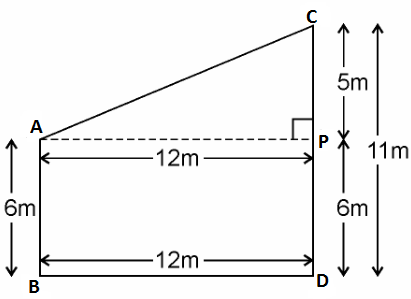Guru

# Two poles of heights 6 m and 11 m stand on a plane ground. If the distance between the feet of the poles is 12 m, find the distance between their tops. Q.12

• 4

How i solve this important question of triangles chapter of class 10th math of exercise6.5 of question no 12 because it an important question of class 10th give me the best way to solve this problem Two poles of heights 6 m and 11 m stand on a plane ground. If the distance between the feet of the poles is 12 m, find the distance between their tops.

Share

1. This answer was edited.

Given, Two poles of heights 6 m and 11 m stand on a plane ground.

And distance between the feet of the poles is 12 m.Let AB and CD be the poles of height 6m and 11m.

Therefore, CP = 11 – 6 = 5m

From the figure, it can be observed that AP = 12m

By Pythagoras theorem for ΔAPC, we get,

AC2 =AP2 + PC2

(12m)2 + (5m)2 = (AC)2

AC2 = (144+25) m= 169 m

AC = 13m

Therefore, the distance between their tops is 13 m.

• 4

• 0